Nothing special here. It’s just a blog post for summarising my algorithm learning course. Although this was already taught in the University, it’s still god to summarize here

# 1. Symbol Tables

Key-value pair abstraction.

• Insert a value with specified key.
• Given a key, search for the corresponding value.

Example

domain name IP address
www.cs.princeton.edu 128.112.136.11
www.princeton.edu 128.112.128.15
www.yale.edu 130.132.143.21
www.harvard.edu 128.103.060.55
www.simpsons.com 209.052.165.60

### Symbol Table APIs

Symbol Tables act as an associative array, associate one value with each key.

# 2. Ordered Symbol Tables

If we order the keys in Symbol table, we can support a much wider number of operations, for example min, max operations and especially range operations.

| operations                    | keys     | values  |
|-------------------------------|----------|---------|
| min() ======================> | 09:00:00 | Chicago |
|                               | 09:00:03 | Phoenix |
| get(09:00:13) ==============> | 09:00:13 | Houston |
|                               | 09:00:59 | Chicago |
|                               | 09:01:10 | Houston |
| floor(09:05:00) ============> | 09:03:13 | Chicago |
|                               | 09:10:11 | Seattle |
| select(7) ==================> | 09:10:25 | Seattle |
|                               | 09:14:25 | Phoenix |
| ============================> | 09:19:32 | Chicago |
| ============================> | 09:19:46 | Chicago |
| keys(09:15:00, 09:25:00) ===> | 09:21:05 | Chicago |
| ============================> | 09:22:43 | Seattle |
| ============================> | 09:22:54 | Seattle |
|                               | 09:25:52 | Chicago |
| ceiling(09:30:00) ==========> | 09:35:21 | Chicago |
|                               | 09:36:14 | Seattle |
| max() ======================> | 09:37:44 | Phoenix |
| size(09:15:00, 09:25:00) = 5  |          |         |
| rank(09:10:25) = 7            |          |         |


# 3. Binary Search Tress

Binary Search Tree is a binary tree in symmetric order. Each node has a key, and every node’s key is

• Larger than all keys in its left subtree.
• Smaller than all keys in its right subtree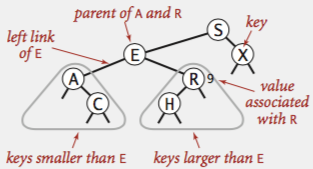Using BST, we can implement all the operations of Ordered Symbol Tables quite efficiently, as long as keys are inserted in random order.

## Node

A Binary Search Tree is a reference to the root Node

class Node {
private Key key;
private Value val;
private Node left, right;
public Node(Key key, Value val) {
this.key = key;
this.val = val;
}
}


## Get method

Return value corresponding to given key, or null if no such key. Traverse from the root node, if less, go left, if greater, go right, if equal, search hit.

public Value get(Key key) {
Node x = root;
while (x != null) {
int cmp = key.compareTo(x.key);
if      (cmp  < 0) x = x.left;
else if (cmp  > 0) x = x.right;
else if (cmp == 0) return x.val;
}
return null;
}


## Insert method

Search for key, then two cases:

• Key in tree ⇒ reset value.
• Key not in tree ⇒ add new node.
private Node put(Node x, Key key, Value val) {
if (x == null) return new Node(key, val);

int cmp = key.compareTo(x.key);
if  (cmp  < 0)
x.left  = put(x.left,  key, val);
else if (cmp  > 0)
x.right = put(x.right, key, val);
else if (cmp == 0)
x.val = val;
return x;
}


## Floor/Ceiling method

Floor: Largest key ≤ a given key

• Case 1: k equals the key at root
• The floor of k is k
• Case 2: k is less than the key at root
• The floor of k is in the left subtree.
• Case 3: k is greater than the key at root
• The floor of k is in the right subtree if there is any key ≤ k in right subtree
• Otherwise it is the key in the root.
private Node floor(Node x, Key key) {
if (x == null) return null;
int cmp = key.compareTo(x.key);

if (cmp == 0)
return x;
if (cmp < 0)
return floor(x.left, key);

Node t = floor(x.right, key);
if (t != null)
return t;
else
return x;
}


Ceiling: the reverse way

## Subtree counts

In each node, we store the number of nodes in the subtree rooted at that node; To implement size(), return the count at the root.

Rank. How many keys < k ?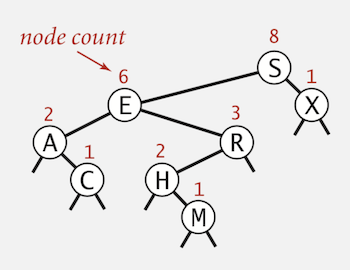public int rank(Key key) {
return rank(key, root);
}

private int rank(Key key, Node x) {
if (x == null) return 0;

int cmp = key.compareTo(x.key);
if      (cmp  < 0)
return rank(key, x.left);
else if (cmp  > 0)
return 1 + size(x.left) + rank(key, x.right);
else if (cmp == 0)
return size(x.left);
}


## In-Order Traversal

• Traverse left subtree.
• Enqueue key.
• Traverse right subtree.
public Iterable<Key> keys() {
Queue<Key> q = new Queue<Key>();
inorder(root, q);
return q;
}

private void inorder(Node x, Queue<Key> q) {
if (x == null) return;

inorder(x.left, q);
q.enqueue(x.key);
inorder(x.right, q);
}


In-Order traversal of a BST yields keys in ascending order

## Lazy Deletion

To remove a node with a given key:

• Set its value to null.
• Leave key in tree to guide search (but don’t consider it equal in search)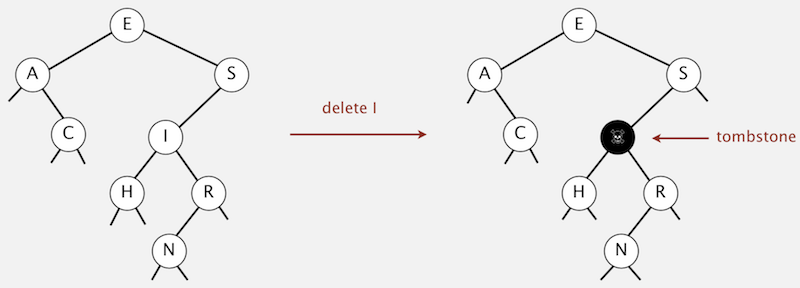Drawback: Tombstone (memory) overload

## Delete the minimum

To delete the minimum key:

• Go left until finding a node with a null left link.
• Replace that node by its right link.
• Update subtree counts.
public void deleteMin() {
root = deleteMin(root);
}

private Node deleteMin(Node x) {
if (x.left == null) return x.right;

x.left = deleteMin(x.left);
x.count = 1 + size(x.left) + size(x.right);
return x;
}


## Delete a specific key using Hibbard deletion

To delete a node with key k: search for node t containing key k.

• Case 0: 0 children Delete t by setting parent link to null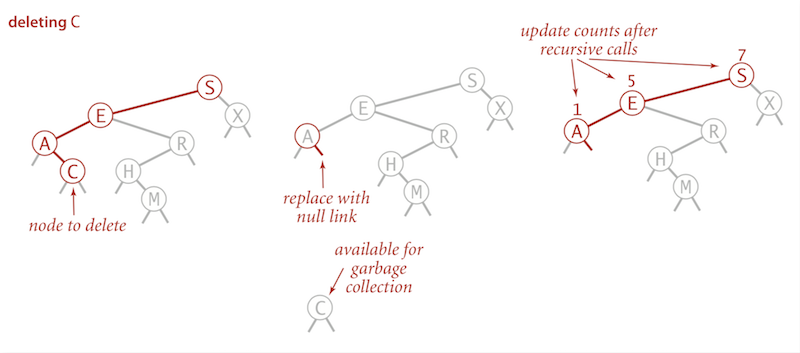• Case 1: 1 child Delete t by replacing parent link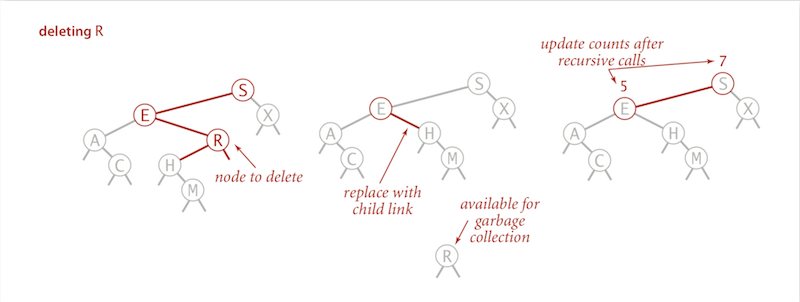• Case 2: 2 children
• Find successor x of t, find the x which can replace the position of the node t (the min of t.right).
• Delete that node x out of the BST using the deleteMin method, but keep the node x in memory.
• Put x in t’s spot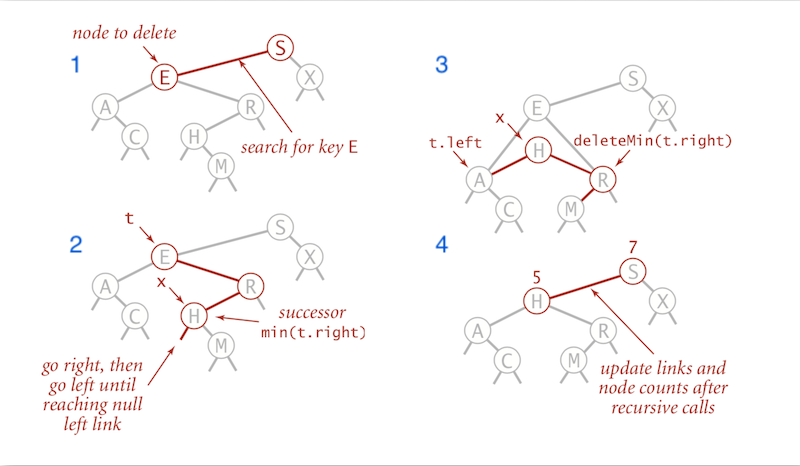public void delete(Key key) {
root = delete(root, key);
}

// delete method returns the new tree after deletion
private Node delete(Node x, Key key) {
if (x == null) return null;

// search for key by traserving left/right
int cmp = key.compareTo(x.key);
if (cmp < 0)
// set the subtree to the new tree after deletion
x.left  = delete(x.left,  key);
else if (cmp > 0)
// set the subtree to the new tree after deletion
x.right = delete(x.right, key);

else {
// case 0 and case 1, return null or the left/right subtree to
// update the link in parent node
if (x.right == null) return x.left;
if (x.left  == null) return x.right;

// case 2, replace with successor
Node t = x;
x = min(t.right);
x.right = deleteMin(t.right);
x.left = t.left;
}

// update subtree counts
x.count = size(x.left) + size(x.right) + 1;
return x;
}